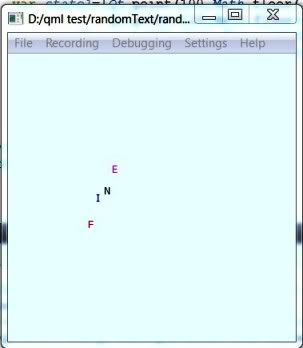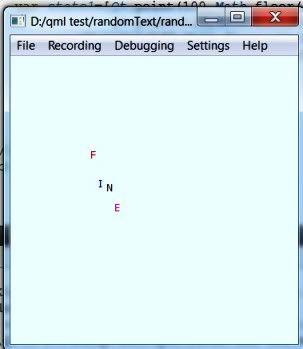# QML-Qt.point-Applications

English

## Introduction

QML Qt is a global object visible in QML and JavaScript namespaces. Note it is not a QML element and no need to be instantiated. It offers useful methods, properties and enumerations from Qt. To use them apply well-known dot notation. In QML documentation the object is categorized in "Utility" category.

In this article we analyze how Qt.point() method could be used to develop dynamic behaviors of QML visible elements and have some "whirl" effects.

The examples bellow are tested in a desktop setting – Win 7, Qt SDK 1.2. The complete snippets are available here.

## Qt.point() Definition

Qt.point() method takes two arguments – x and y coordinates (in screen area) - and returns a point  :

```point Qt::point(int x, int y)<code>

The type ''point'' is a QML [http://doc.qt.io/qt-4.8/qdeclarativebasictypes.html basic] type. A ''point'' type could be initialized in two ways – as a string or calling ''Qt.point():''

<code>Rectangle {
width: 360
height: 360
property variant propertyTest: "30,40"
property variant anotherTest: Qt.point(100,200)
Component.onCompleted: {
console.log("This is a string definition",propertyTest.x)
console.log("This is a Qt.point()definition",anotherTest.y)
}
}
```

We recall that the variant type property accepts point type values. The property propertyTest (anotherTest) is of point type, has two attributes (x and y) and which are accessible through the dot notation.

Note the difference in the x and y definition in Qt.point() and an Item element for example. In the first case x and y arguments are of int type whereas in an Item definition they are of real type.

## Carousel Sample

There is a nice carousel example, which use PathView element in implementation. We will demonstrate the similar effects using Qt.point() method.

The idea is as follows. A rectangle will be moved over a predefined trajectory, which is described as a sequence of Qt.point() points. The rectangle to be moved is defined as a component file – RunArea.qml. The points sequence is stored in a JavaScript array – variable path. A timer periodically fires an onTrrigered signal after which the rectangle is moved to a new position. Firstly, the Loader test loads the component RunArea.qml and then changes its position.

```Item{
width:400;height:400
property int ind:0
Timer {
interval: 500; running: true; repeat: true
onTriggered: {
var path=[Qt.point(100,30),Qt.point(130,60),Qt.point(160,90),
Qt.point(130,120),Qt.point(100,150),Qt.point(70,120),
Qt.point(40,90),Qt.point(70,60)]
test.source="RunArea.qml"
test.item.x=path[ind].x
test.item.y=path[ind].y
ind=ind+1
if(ind==8)
ind=0
}
}
}
```

## Whirling Text

We will animate a text (the word COOL) rotating it around an origin. Each symbol of the text is putted in a rectangle (rec1, rec2, rec3, rec4) and controlled individually. To get an access to color property of the Text element for each rectangle we define an alias col. The collection of these rectangles is stored in property cloud that is of type list. Five states are defined and they map five different positions of the text symbols. In the programming code these states are represented by JavaScript variables state1, state2, state3, state4 and state5.

The changes of the states are initiated by a Timer element. Each state is composed by QML points stored in corresponding state variables. You may experiment altering interval property of the Timer element. Additional effects you could have changing the color property of the rectangles – in the snippets it is commented.

```Rectangle {
width: 360
height: 360
property int ind:0
Rectangle {id:rec1;x:100; y:100;width:20;height:20;
// color:"yellow"
property alias col:symbol1.color
Text {id:symbol1;text:"C"}
}
Rectangle {id:rec2;x:110;y:100;width:20;height:20;
// color:"yellow"
property alias col:symbol2.color
Text {id:symbol2;text:"O"}
}
Rectangle {id:rec3;x:120;y:100;width:20;height:20;
// color:"yellow"
property alias col:symbol3.color
Text {id:symbol3;text:"O"}
}
Rectangle {id:rec4;x:130;y:100;width:20;height:20;
// color:"yellow"
property alias col:symbol4.color
Text {id:symbol4;text:"L"}
}
property list<Item> cloud
Timer {
interval: 100; running: true; repeat: true
onTriggered: {
cloud=[rec1,rec2,rec3,rec4]
var state1=[Qt.point(100,100),Qt.point(110,90),Qt.point(120,80),
Qt.point(130,70)]
var state2=[Qt.point(100,100),Qt.point(110,110),Qt.point(120,120),
Qt.point(130,130)]
var state3=[Qt.point(100,100),Qt.point(100,120),Qt.point(100,140),
Qt.point(100,160)]
var state4=[Qt.point(100,100),Qt.point(90,120),Qt.point(80,140),
Qt.point(70,160)]
var state5=[Qt.point(100,100),Qt.point(90,80),Qt.point(80,60),
Qt.point(70,40)]
var temp;
if(ind0)
{
temp=state1
for(var i1=0;i1<=3;i1=i1+1) {
cloud[i1].x=temp[i1].x
cloud[i1].y=temp[i1].y
cloud[i1].col="red"
}
ind=ind+1;
}
else if(ind1)
{
temp=state2
for(var i2=0;i2<=3;i2=i2+1){
cloud[i2].x=temp[i2].x
cloud[i2].y=temp[i2].y
}
ind=ind+1
}
else if(ind2)
{
temp=state3
for(var i3=0;i3<=3;i3=i3+1){
cloud[i3].x=temp[i3].x
cloud[i3].y=temp[i3].y
cloud[i3].col="purple"
}
ind=ind+1
}
else if(ind3)
{
temp=state4
for(var i4=0;i4<=3;i4=i4+1){
cloud[i4].x=temp[i4].x
cloud[i4].y=temp[i4].y
cloud[i4].col="darkmagenta"
}
ind=ind+1
}
else if(ind==4)
{
temp=state5
for(var i5=0;i5<=3;i5=i5+1){
cloud[i5].x=temp[i5].x
cloud[i5].y=temp[i5].y
cloud[i5].col="navy"
}
ind=0
}
else {console.log("error")}
}
}
}
```

## Generating of Random Trajectories

Now we want to change the coordinates of the symbols of the word "FINE" in a random manner. For simplicity we assume that only y coordinates will be changed with a random offset. The random numbers we get with the JavaScript function random(). It generates random numbers (of type real) in the interval (0,1). With the JavaScript function floor() we round the returned value from random() to its downwards nearest integer. The both functions are methods of the JavaScript object Math so we call them like that - Math.random() and Math.floor(). We could use a normalizing factor to extend the interval of returned random numbers as is illustrated in the next line:

```Math.floor((Math.random()*10)+1)
```

The above call returns numbers between 1 and 10.

The point is if the method Qt.point() accepts functions calls as arguments like that Qt.point(Math.floor(Math.random()),Math.floor(math.random()))? The next code fragment gives a positive answer to this question:

```//The next code fragment tests function substitution
Item {width:400;height:400
property variant test:Qt.point(30,getPoint())

//The call to getPoint()is used as Qt.point() argument
function getPoint()
{
return 200
}

Rectangle { id:dummy
Component.onCompleted: {
console.log("testY=",test.y)
}
}
}//end Item
```

The code implementation of the "twisted" word uses the same QML constructs as in the previous section. It is as follows:

```import QtQuick 1.1
Rectangle {
width: 360
height: 360
color:"azure"
Rectangle {id:rec1;x:100; y:100;width:20;height:20;
color:"transparent"
property alias col:symbol1.color
Text {id:symbol1;text:"F";color:"red"}
}
Rectangle {id:rec2;x:110;y:100;width:20;height:20;
color:"transparent"
property alias col:symbol2.color
Text {id:symbol2;text:"I";color:"blue"}
}
Rectangle {id:rec3;x:120;y:100;width:20;height:20;
color:"transparent"
property alias col:symbol3.color
Text {id:symbol3;text:"N";color:"black"}
}
Rectangle {id:rec4;x:130;y:100;width:20;height:20;
color:"transparent"
property alias col:symbol4.color
Text {id:symbol4;text:"E";color:"magenta"}
}
property list<Item> cloud
Timer {
interval: 1000; running: true; repeat: true
onTriggered: {
cloud=[rec1,rec2,rec3,rec4]
var state1=[Qt.point(100,Math.floor((Math.random()*100)+10)),
Qt.point(120,Math.floor((Math.random()*100)+10)),
Qt.point(140,Math.floor((Math.random()*100)+10)),
Qt.point(160,Math.floor((Math.random()*100)+10))
]
for(var i1=0;i1<=3;i1=i1+1) {
cloud[i1].y=state1[i1].y+100
}//end for
}//end of onTriggered
}//end Timer
}
```

The next screenshots illustrate the running code: